﻿ 基于Logistic混沌映射的比特级数字图像加密算法研究 Research on Bit-Level Digital Image Encryption Algorithm Based on Logistic Chaotic System

Journal of Image and Signal Processing
Vol.05 No.03(2016), Article ID:17971,7 pages
10.12677/JISP.2016.53012

Research on Bit-Level Digital Image Encryption Algorithm Based on Logistic Chaotic System

Jingjing Huang, Qinghua Wang, Zhenhua Li

Nanjing University of Science and Technology, Nanjing Jiangsu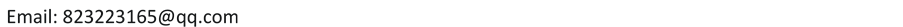Received: Jun. 25th, 2016; accepted: Jul. 10th, 2016; published: Jul. 13th, 2016ABSTRACT

A bit-level digital image encryption method based on Logistic chaotic system is proposed. Each pixel value of a grayscale image uses eight-bit binary numbers, and the two-dimensional gray image is converted to a one-dimensional string containing 0 and 1. We generate the Logistic chaotic sequence with the given initial value, and then sort the chaotic sequence to obtain a location index. According to the location index, the original image is scrambled in bit-level. The pixels fusion and pixel scrambling encryption of an image are realized simultaneously. Experiments show that the algorithm is simple, safe and efficient.

Keywords:Chaos, Scrambling, Image Encryption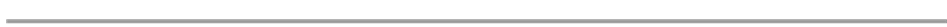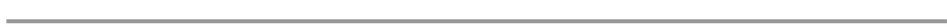1. 引言

2. Logistic映射

Logistic映射是一种应用十分广泛的动力系统，其一般定义形式如下  ：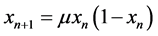(1)

3. 加密解密算法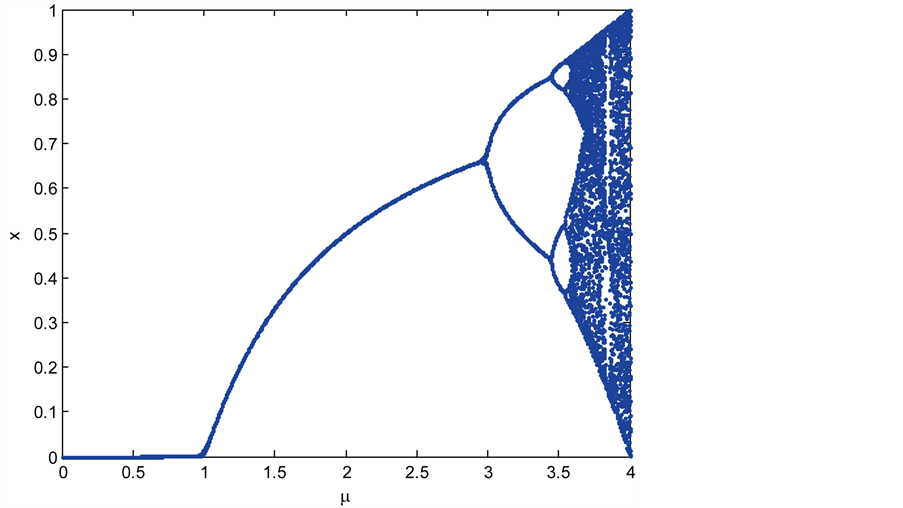Figure 1. The bifurcation diagram of Logistic mapping(2)

4. 加密解密数值实验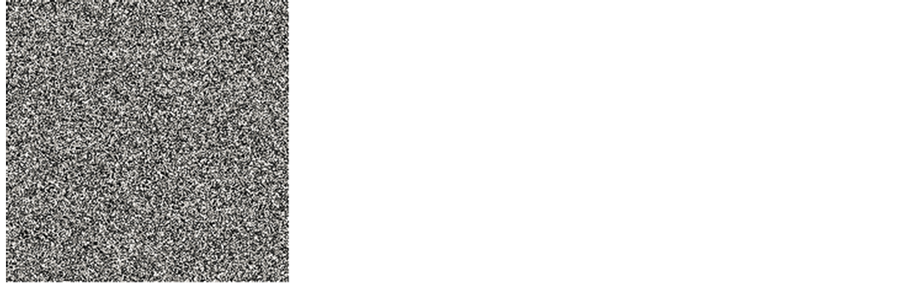(a) 原图像 (b) 加密图像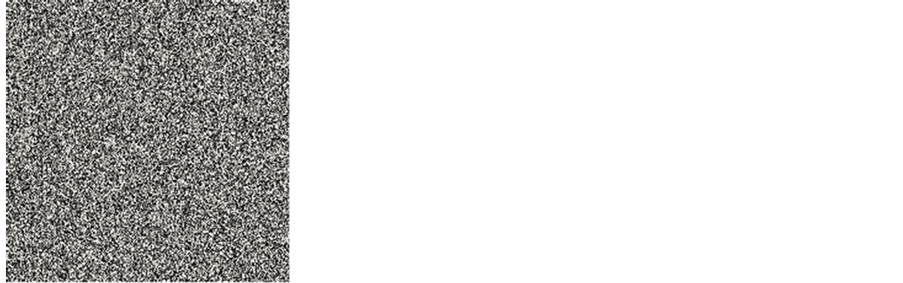(c1) 正确解密图像 (c2) 错误解密图像 (c3) 错误解密图像

Figure 2. Encrypted image obtained with a different key to decrypt

5. 实验结果及安全性分析

5.1. 密钥空间和雪崩效应分析

5.1.1. 密钥空间分析

5.1.2. 密钥雪崩效应分析

5.2. 抗统计攻击

5.2.1. 直方图分析Figure 3. Ciphertext sensitivity to key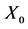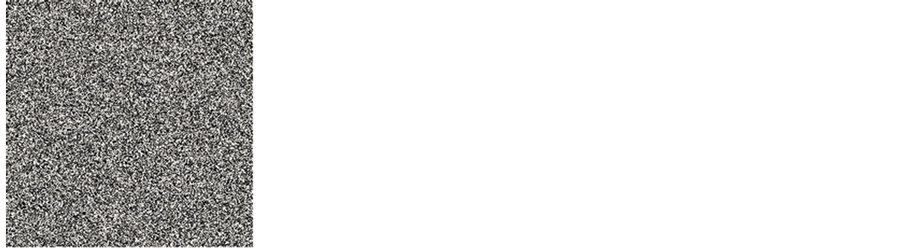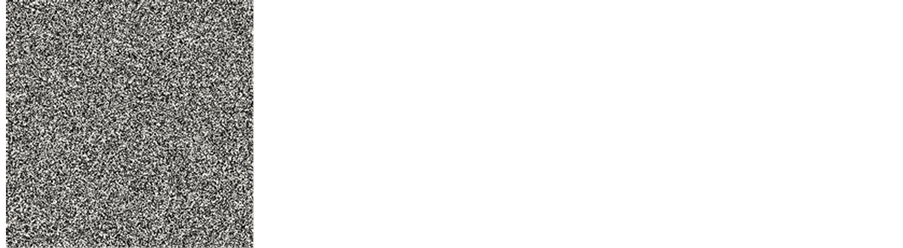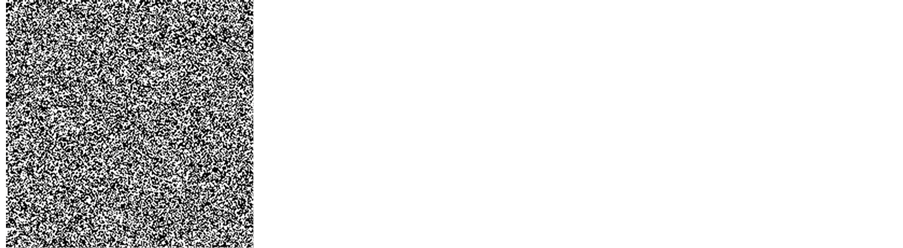Figure 4. Ciphertext sensitivity to keyFigure 5. The gray value histogram of the images encrypted before and after

5.2.2. 相关性分析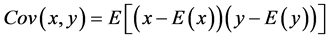(3)(4)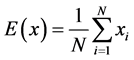(5)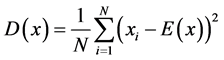(6)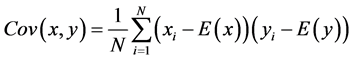(7)

6. 结束语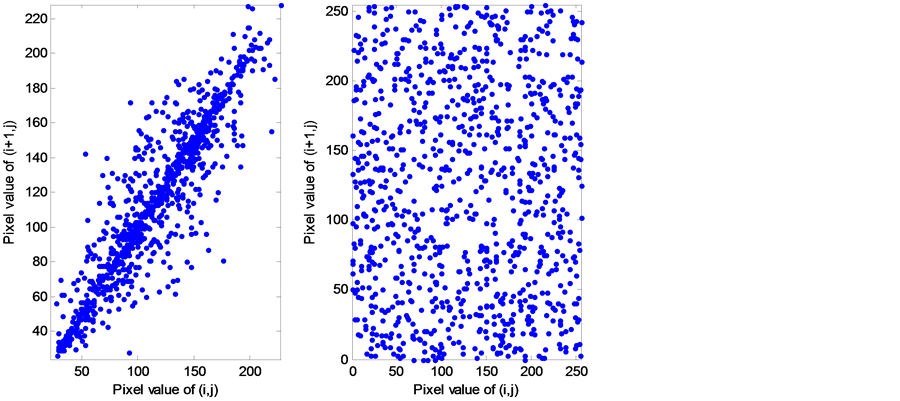Figure 6. The correlation analysis between adjacent pixels of plaintext and ciphertextTable 1. The correlation coefficient between adjacent pixels of plaintext and ciphertext

Research on Bit-Level Digital Image Encryption Algorithm Based on Logistic Chaotic System[J]. 图像与信号处理, 2016, 05(03): 88-94. http://dx.doi.org/10.12677/JISP.2016.53012

1. 1. 王朋飞, 冯桂. 基于混沌动力系统的数字图像加密方法[J]. 计算机工程与应用, 2007, 43(13): 55-57.

2. 2. 陈关荣. 动力系统的混沌化[M]. 上海: 上海交通大学出版社, 2006.

3. 3. 张永红, 张博. 基于Logistic混沌系统的图像加密算法研究[J]. 计算机应用研究, 2015, 32(6): 1770-1773.

4. 4. 李彩虹, 李贻斌, 赵磊, 等. 一维Logistic映射混沌伪随机序列统计特性研究[J]. 计算机应用研究, 2014, 31(5): 1403-1406.

5. 5. Chen, J.X., Zhu, Z.L., Fu, C., et al. (2013) An Improved Permutation-Diffusion Type Image Cipher with a Chaotic Orbit Perturbing Mechanism. Optics Express, 21, 27873-27890. http://dx.doi.org/10.1364/OE.21.027873

6. 6. 黄润生. 混沌及其应用[M]. 武汉: 武汉大学出版社, 2000.

7. 7. Fu, C., Chen, J.J., Zou, H., et al. (2012) A Chaos-Based Digital Image Encryption Scheme with an Improved Diffusion Strategy. Optics Express, 20, 2363-2378. http://dx.doi.org/10.1364/OE.20.002363

8. 8. Wong, K.W., Kwok, S.H. and Law, W.S. (2006) A Fast Image Encryption Scheme Based on Chaotic Standard Map. Physics Letters A, 372, 2645-2652. http://dx.doi.org/10.1016/j.physleta.2007.12.026